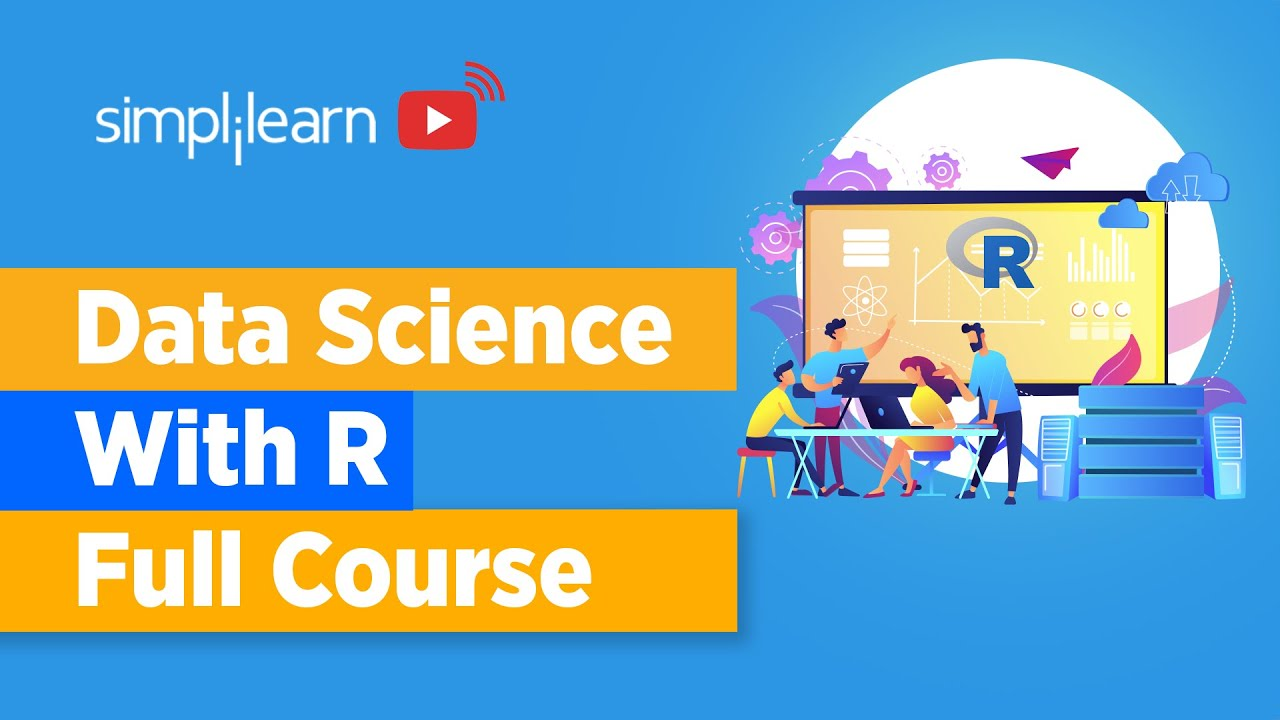# Data Science With R Full Course | Learn Data Science With R | Data ScienceIn this video on Data Science with R full course, we’ll start by learning data science from an animated video, essential concepts in data science, important packages in R, data science algorithms such as Linear regression, logistic regression, decision trees, random forest, including time-series analysis. Finally, you will get an idea about the Salary structure, Skills, Jobs, and resume of a data scientist.

In this video on Data Science with R full course, we’ll start by learning data science from an animated video, essential concepts in data science, important packages in R, data science algorithms such as Linear regression, logistic regression, decision trees, random forest, including time-series analysis. Finally, you will get an idea about the Salary structure, Skills, Jobs, and resume of a data scientist.

## Data Science With Python Training | Python Data Science Course | Intellipaat

🔵 Intellipaat Data Science with Python course: https://intellipaat.com/python-for-data-science-training/In this Data Science With Python Training video, you...

## R For Data Science Full Course | Data Science With R Full Course |Data Science Tutorial

Learn the essential concepts in data science and understand the important packages in R for data science. You will look at some of the widely used data science algorithms such as Linear regression, logistic regression, decision trees, random forest, including time-series analysis. Finally, you will get an idea about the Salary structure, Skills, Jobs, and resume of a data scientist.

## 5 Examples to Compare Python Pandas and R data.table

In this tutorial, we'll learn 5 Examples to Compare Python Pandas and R data.table. Read through this article and see which one is better for your project.

## Must-Know Data Science Libraries in Python

In this tutorial, we'll learn Must-Know Data Science Libraries in Python. Python is the most widespread and popular programming language in data science, software development, and related fields. The simplicity of…

## How To Build A Data Science Career In 2021

In Conversation With Dr Suman Sanyal, NIIT University,he shares his insights on how universities can contribute to this highly promising sector and what aspirants can do to build a successful data science career.